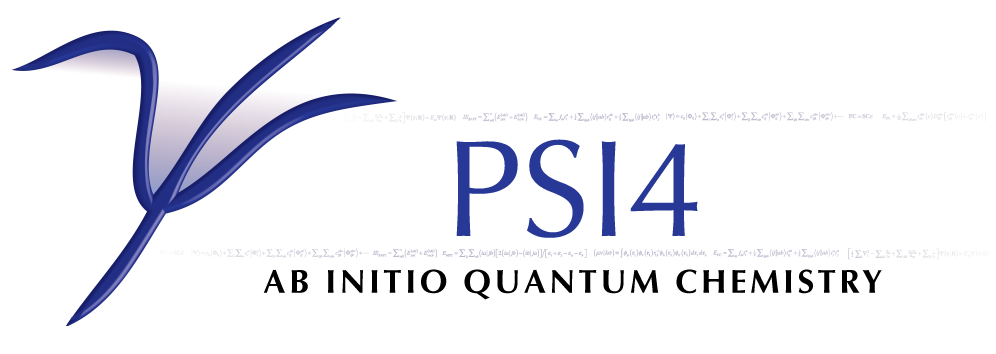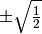# MCSCF¶

Performs RHF/UHF/ROHF/TCSCF and more general MCSCF computations. Called as the starting point for multireference coupled cluster computations.

## General¶

### CANONICALIZE_ACTIVE_FAVG¶

Do canonicalize the active orbitals such that the average Fock matrix is diagonal?

### CANONICALIZE_INACTIVE_FAVG¶

Do canonicalize the inactive (DOCC and Virtual) orbitals such that the average Fock matrix is diagonal?

### CI_DIIS¶

Do use DIIS extrapolation to accelerate convergence of the CI coefficients?

### DIIS¶

Do use DIIS extrapolation to accelerate convergence of the SCF energy (MO coefficients only)?

### DIIS_MAX_VECS¶

Maximum number of error vectors stored for DIIS extrapolation

• Type: integer
• Default: 7

### DOCC¶

The number of doubly occupied orbitals, per irrep

• Type: array
• Default: No Default

### D_CONVERGENCE¶

Convergence criterion for density.

### E_CONVERGENCE¶

Convergence criterion for energy.

### FAVG¶

Do use the average Fock matrix during the SCF optimization?

### FAVG_START¶

Iteration at which to begin using the averaged Fock matrix

• Type: integer
• Default: 5

### FOLLOW_ROOT¶

Which solution of the SCF equations to find, where 1 is the SCF ground state

• Type: integer
• Default: 1

### FORCE_TWOCON¶

Do attempt to force a two configruation solution by starting with CI coefficents of?

Do ?

### LEVEL_SHIFT¶

Level shift to aid convergence

• Type: double
• Default: 0.0

### MAXITER¶

Maximum number of iterations

• Type: integer
• Default: 100

Do read in from file the MOs from a previous computation?

### REFERENCE¶

Reference wavefunction type

• Type: string
• Possible Values: RHF, ROHF, UHF, TWOCON, MCSCF, GENERAL
• Default: RHF

### SOCC¶

The number of singly occupied orbitals, per irrep

• Type: array
• Default: No Default

### TURN_ON_ACTV¶

• Type: integer
• Default: 0

### WFN_SYM¶

The symmetry of the SCF wavefunction.

• Type: string
• Possible Values: A, AG, AU, AP, APP, A1, A2, B, BG, BU, B1, B2, B3, B1G, B2G, B3G, B1U, B2U, B3U, 0, 1, 2, 3, 4, 5, 6, 7, 8
• Default: 1

## Expert¶

### ROTATE_MO_ANGLE¶

For orbital rotations after convergence, the angle (in degrees) by which to rotate.

• Type: integer
• Default: 0

### ROTATE_MO_IRREP¶

For orbital rotations after convergence, irrep (1-based, Cotton order) of the orbitals to rotate.

• Type: integer
• Default: 1

### ROTATE_MO_P¶

For orbital rotations after convergence, number of the first orbital (1-based) to rotate.

• Type: integer
• Default: 1

### ROTATE_MO_Q¶

For orbital rotations after convergence, number of the second orbital (1-based) to rotate.

• Type: integer
• Default: 2